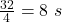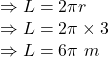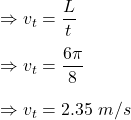Question

A dog is running in a circle around a tree, trying to catch a rabbit. The dog’s owner notices the
dog completes 4 laps around the tree in 32 seconds and the radius of the dog’s circular path is
3 meters. What is the tangential velocity of the dog?

1.quangkhai

Explanation:

Given

Dog completes 4 laps around the tree in  32 seconds

Radius of the circular path is 3 m

Time period for single lap isLength of the track is equivalent to the perimeter of the pathTangential velocity is given byThus, the tangential velocity is 2.35 m/s